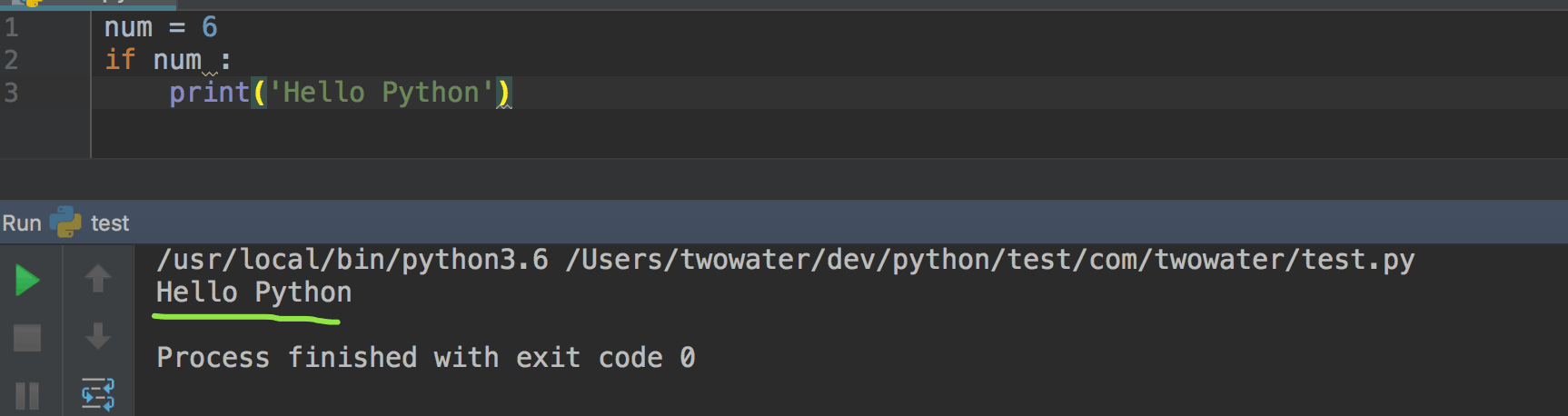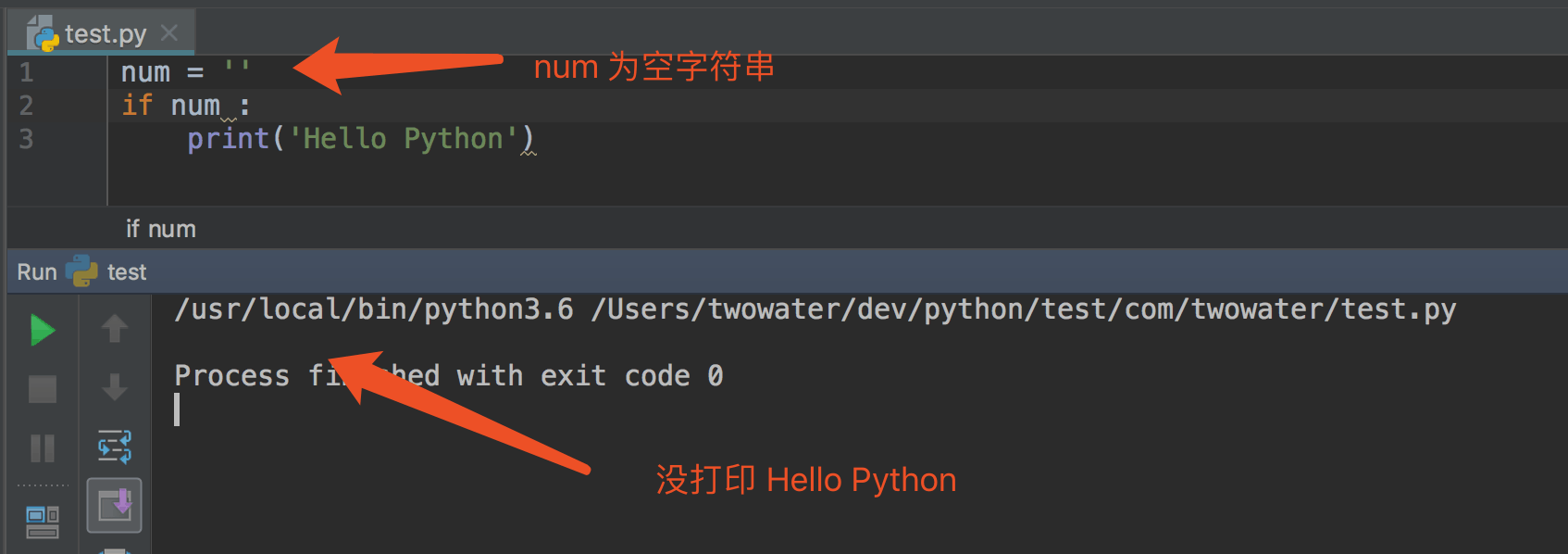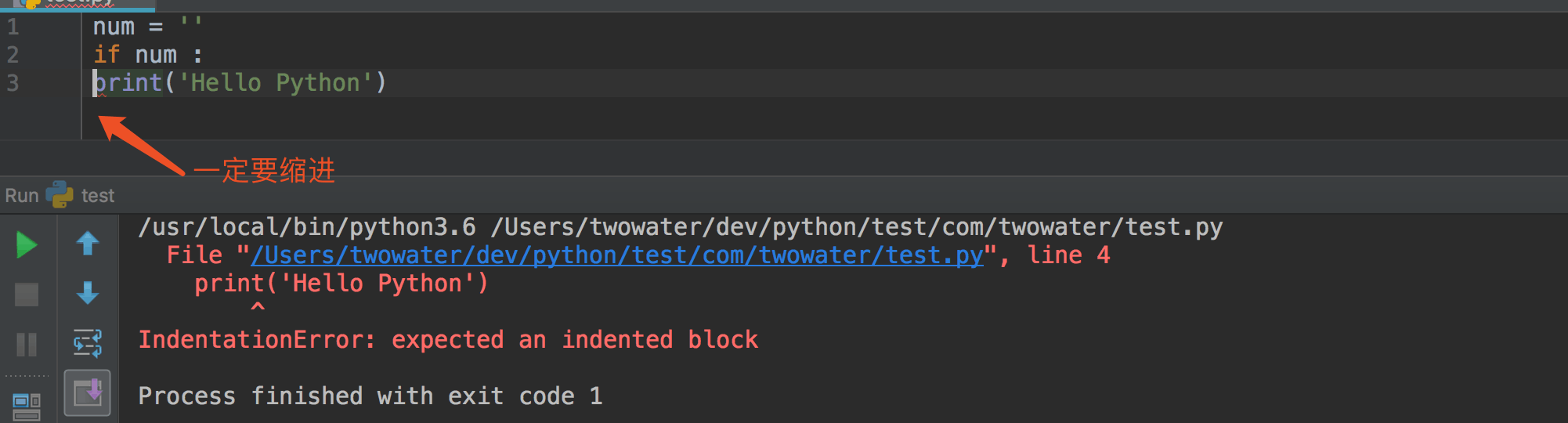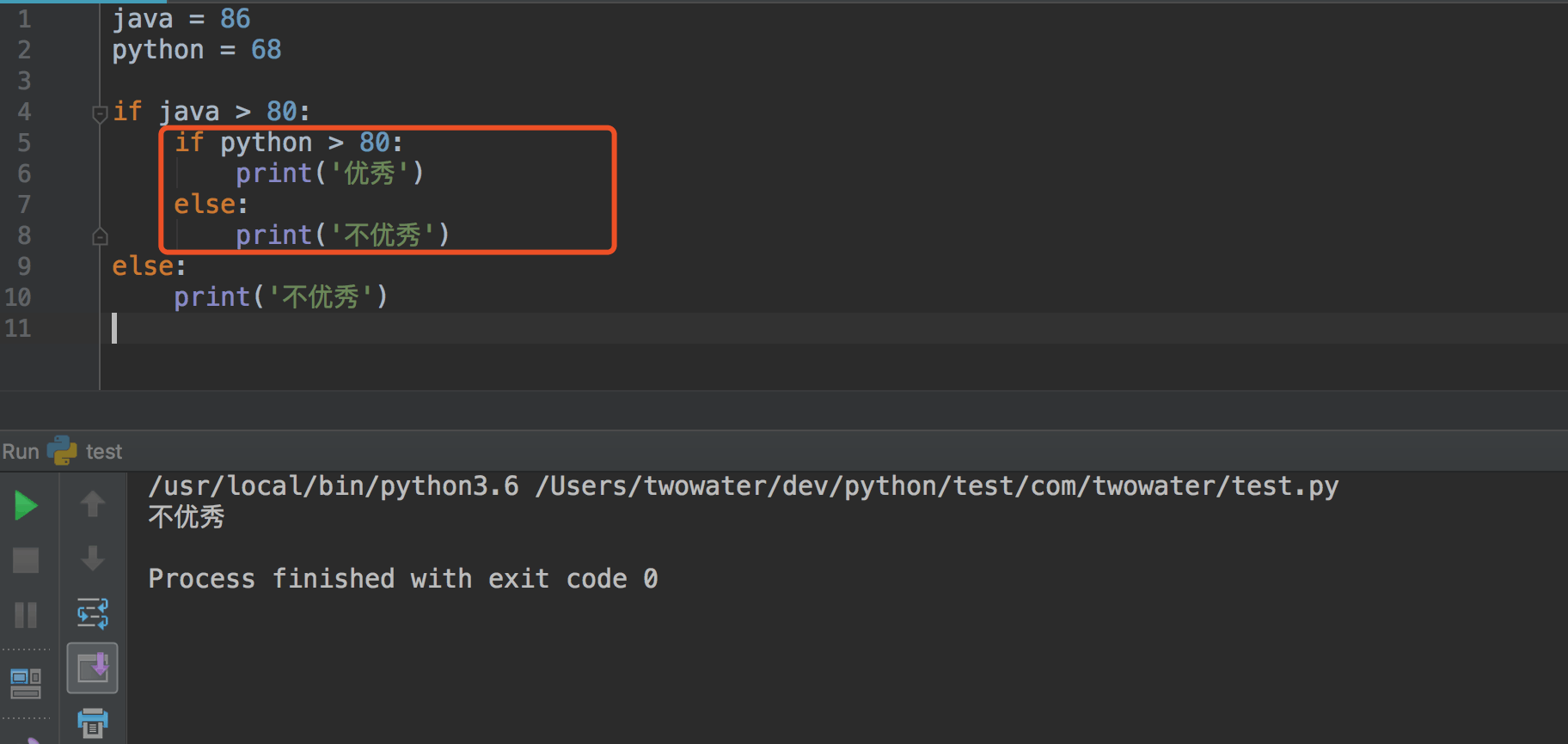# 一、条件语句

## 1、什么是条件语句

Python 条件语句跟其他语言基本一致的，都是通过一条或多条语句的执行结果（ True 或者 False ）来决定执行的代码块。

Python 程序语言指定任何非 0 和非空（null）值为 True，0 或者 null 为 False。## 2、if 语句的基本形式

Python 中，if 语句的基本形式如下：

``````if 判断条件：
执行语句……
else：
执行语句……
``````

if 语句的判断条件可以用>（大于）、<(小于)、==（等于）、>=（大于等于）、<=（小于等于）来表示其关系。

``````# -*-coding:utf-8-*-

results=59

if results>=60:
print ('及格')
else :
print ('不及格')

``````

``````不及格
``````

``````num = 6
if num :
print('Hello Python')
``````## 3、if 语句多个判断条件的形式

``````if 判断条件1:
执行语句1……
elif 判断条件2:
执行语句2……
elif 判断条件3:
执行语句3……
else:
执行语句4……
``````

``````# -*-coding:utf-8-*-

results = 89

if results > 90:
print('优秀')
elif results > 80:
print('良好')
elif results > 60:
print ('及格')
else :
print ('不及格')

``````

``````良好
``````

## 4、if 语句多个条件同时判断

or （或）表示两个条件有一个成立时判断条件成功

and （与）表示只有两个条件同时成立的情况下，判断条件才成功。

``````# -*-coding:utf-8-*-

java = 86
python = 68

if java > 80 and  python > 80:
print('优秀')
else :
print('不优秀')

if ( java >= 80  and java < 90 )  or ( python >= 80 and python < 90):
print('良好')

``````

``````不优秀

``````

## 5、if 嵌套

if 嵌套是指什么呢？